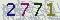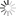﻿ 数学学院珠峰论坛第474期：Romanoff's theorem for polynomials over finite fields revisited-山东大学新闻网### 数学学院珠峰论坛第474期：Romanoff's theorem for polynomials over finite fields revisited

Romanoff's theorem for polynomials over finite fields revisited

Let g be a given polynomial of positive degree over a finite field. Shparlinski and Weingartner proved that the proportion of monic polynomials of degree n which can be represented by \$h + g^k\$ has the order of magnitude 1/deg g, where h is chosen from the setof irreducible monic polynomials of degree n and k∈N. In this talk,we show that the proportion of monic polynomials of degree n which can be written as \$l + g^p\$ where l is the product of two monic irreducible polynomials with deg l = n and p is a prime number, still has the order of magnitude 1/deg g.

12月17日（周五）15:00

【作者：张志越    来自：数学学院    编辑：新闻网工作室    责任编辑：蒋晓涵 　】

 验证码看不清楚,换张图片 共0条评论    共1页   当前第1页 拖动光标可翻页查看更多评论

### 最新发布### 新闻排行• 山大日记
• 山大人物
• 视点微信
• 互动话题
• 视点图志
• 精彩视频